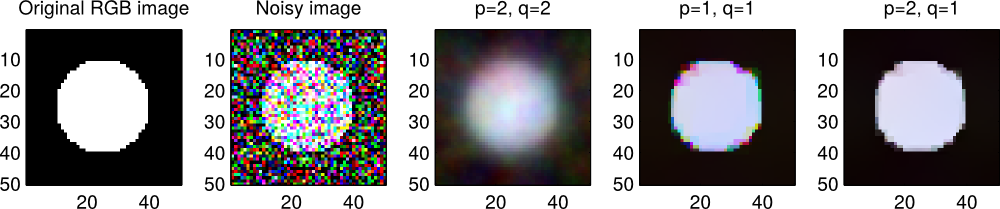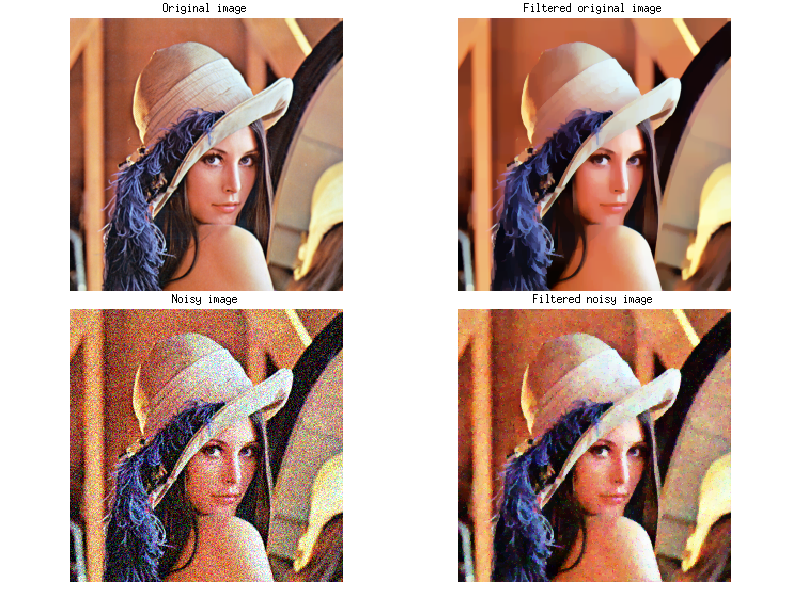# Rémi Flamary

Professional website

## Total variation in images

$\def\x{\mathbf{x}} \def\X{\mathbf{X}} \def\Y{\mathbf{Y}}$

In this demo, we illustrate the effect of Total Variation regularization on images and how it can be used for image denoising.

### Short introduction to Total Variation (TV)

#### Definition

Total variation is a measure of the complexity of an image with respect to its spatial variation. It has several variations in the image processing litterature. In this demo we will discuss its extension to color images. In color images, one can consider each pixel $\x\in\mathbb{R}^3$ as a 3D vector.

The total variation of a color image $\X$ can be expressed as

$$\begin{equation*} TV(\X)=\sum_{i,j\in\mathcal{N}} \|\x_i-\x_j\|_p^q \end{equation*}$$

where $\mathcal{N}$ defines the pixel neighborhood (usually the horizontal and vertical adjacent pixels) and $\|\cdot\|_p^q$ is the $\ell_p$ norm to the power of $q$.

We will focus in the following to 3 special cases

• Classical $\ell_1$ TV computed independently on each color component.
\begin{align*} \|\x\|_1=\sum_k\|\x_k\|_1&\quad\;\; (p=1,q=1) \end{align*}
• $\ell_2$ TV computes the euclidean norm of the vector.
\begin{align*} \|\x\|_2=\left(\sum_k\x_k^2\right)^{\frac{1}{2}}&\;\;\; (p=2,q=1) \end{align*}
• Squared $\ell_2$ TV computes the squared euclidean norm of the vector.
\begin{align*} \|\x\|_2=\sum_k\x_k^2&\quad\quad\quad (p=2,q=2) \end{align*}

Those variants of TV measure different spatial variation. $\ell_1$ wil sum the absolute values of the finite differences for each color component independently. $\ell_2$ and squared $\ell_2$ will sum respectively the norm and squared norm of the vector of difference treating all the color components simultaneously.

#### TV denoising

TV can be also be used for image denoising. This can be done by computing the following optimization problem

\begin{align*} \min_\X\quad \|\X-\Y\|^2+\lambda TV(\X) \end{align*}

wher $\lambda$ is a positive regularization term and $\Y$ is the noisy image. This optimization find the image $\X$ thet both fit the noisy image but also has a limited TV. An example of the result of this optimization is given in the following image.In this image, we can clearly see that the squared $\ell_2$ TV leads to a smooth image at the cost of loosing sharp edges and details in the image. $\ell_1$ and $\ell_2$ TV both keep sharp edges. in the case of $\ell_1$ TV, all color component are treated independently leading to color artifacts around the edges.

### Regularization demo

In order to see the effect of TV regularization on a real-life image, we will use the widly known Lena picture. We will apply the regularization on both the original image (up) and an image that contains noise (down).

Note that due to the size of the images, the update might take a while when changing the parameters, depending on your internet provider.#### Performance

l1 norm
l2 norm
l2 norm squared

Current value: 0.07

Signal to noise ratio

SNR = 18.99 dB

Both the TV variant and the regularization parameter $\lambda$ can be changed in this demo. The Signal to noise ratio of the reconstructed image is also provided.

Note that an early stopping is used which might return an approximate solution for some values of the regularization parameter.

### References

Total Variation have been around in the image processsing comunity for a while now since the pioneer works of . A nice and simple paper about the extension to color image cen be found in . Finally the algorithm used for solving the optimization probleme is a variant of ADMM as discussed in .

 Nonlinear total variation based noise removal algorithms, Rudin, L. I.; Osher, S.; Fatemi, E. (1992). Physica D 60: 259–268.

 A generalized vector-valued total variation algorithm., Rodriguez Paul, and Brendt Wohlberg. Image Processing (ICIP), 2009 16th IEEE International Conference on. IEEE, 2009.

 An ADMM algorithm for a class of total variation regularized estimation problems, Wahlberg, B., Boyd, S., Annergren, M., & Wang, Y. (2012).